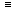# Aptitude - Chain Rule - Discussion

### Discussion :: Chain Rule - General Questions (Q.No.12)

12.

In a camp, there is a meal for 120 men or 200 children. If 150 children have taken the meal, how many men will be catered to with remaining meal?

 [A]. 20 [B]. 30 [C]. 40 [D]. 50

Explanation:

There is a meal for 200 children. 150 children have taken the meal.

Remaining meal is to be catered to 50 children.

Now, 200 children120 men.

 50 children120 x 50= 30 men. 200

 Saranya said: (Feb 4, 2011) Why 120/200? please explain.

 Avijeet said: (Sep 4, 2011) @ seranya-- its given that 200 children= 120 men So 1 child= 120/200 men hence, 150 child=120/200*150= 90 men. so meal will be available for 120-90= 30 men

 Chalam said: (Dec 27, 2011) It's simple like this. If 5 pens cost 25 Rs 1 pen cost? 25/5 = 5.

 Hemanth said: (Feb 3, 2012) In this type of problems how to find the proportionality (Direct or Indirect) ?

 Geethu said: (Mar 28, 2012) Ya its eazy.... 200 children = 120 men 1 children = 120/200 men we have food remaining for 50 children So, 50 children = 120/200*50 = 30 men

 Satish said: (Apr 5, 2012) 200 children= 120 men ---- (1). 50 children= 30 men ---- (2). 150 children takes the food, so there is food for only 50 children from 2. 30 men will be catered the remaining meal.

 Rukhsar said: (Nov 3, 2013) 1 men can have food = 1/120. 1 child can have food = 1/200. 150 child can have food = 150*1/200 = 150/200. Left food = 1-150/200 = 50/200. 1/120 portion of the food taken by = 1 men. 50/200 portion of the food taken by = 1/(1/120)*(5/20) = 30.

 Macdon said: (Feb 20, 2014) 200 children = 120 men. 1 child = 120/200. = 0.6. Hence, 50 children - 0.6*50 = 30 men.

 Bbc said: (Aug 31, 2014) They are just asking how many people are equal to 50 children. 120:x = 200:50. x = (120*50)/200 = 30.

 Indiabix said: (Sep 9, 2014) 200c = 120m. => 100c = 60m. =>50c = 30m. Out of 200c, 150c have taken meal. So, remaining food is for 50c which is equal to 30m.

 Ram said: (Jun 11, 2015) Let man = m and children = y. Then meal of 120m = 200c. Meal of 1m = (200/120)c. 1m = 5/3c. i.e (5/3)c = 1m. 5c = 3m. 1c = (3/5) m. 50c = (3/5*50) m. 50c = 30m.

 Ashok said: (Jun 27, 2015) Ratio for 120 and 200 = 3:5. Then, 150/5 = 30. So 1 part = 30, Then 3 parts for men is 3*30 = 90 and 5 parts for children is 5*30 = 150. So 90 men = 150 children. Remaining men = 30.

 Bindubrisk said: (Mar 17, 2016) It's so simple here 120:200 is nothing but 3:5 means 3 men =5 children means 1 child=3/5 men and remaining 50 children=50* (3/5) =30 and its the answer it is easy.

 Aku said: (Jul 15, 2016) @Hemanth proportionality is clearly visible, more men catered imply more children catered there is direct proportionality. i.e 120 : x :: 200 : 150. 200 * x = 150 * 120. x = 90 so, 150 children are equivalent to 90 men, remaning food is for men = 120 - 90 = 30.

 Farhaaz said: (Sep 27, 2016) The solution to this type of question can be found from observation. Given that 150 children out of 200 children have taken the meal.i.e, 3/4th of children have been supplied by the meal. So 1/4 the of the children have to be supplied with the remaining meal. As it is given that 120 men = 200 children. Therefore the remaining meal must be supplied to 1/4th of 120 men viz.,30 men.

 Sowmiya said: (Aug 30, 2017) How 120/200? Please explain.

 Zoizo said: (Jul 3, 2018) Shouldn't it be 200*120/50?

 Saiganesh Murarishetty said: (Aug 25, 2018) Here; Remaining children are 50 and this is 25% of 200, so 25% of men is 30.

 Atul said: (Nov 13, 2019) 120/200 = men : children. 15men = 25 children. 200-150 = 50(consumed). (25x2)Children = 50(remaning). :.(15x2)men = 30(remaining).